### Daniel's Ethiopic Number Algorithm #4

For one reason or another during the last four years it seems necessary that each year I write a new number algorithm for converting Arabic numbers into Ethiopic. Usually this happens as a memory test that fails and then a new algorithm is created and new insight gained. This year I was finally getting around to HTMLing last year's algorithm but couldn't find it! I tried to rediscover it from some computer code but ended up inventing yet another algorithm.

I think this one is pretty simple, I've tried to emphasize that in the presentation below. The key is that numbers are read in groups of 2 and each group gets the same conversion process. There is only a single special rule that is discussed at the end:

 1) 7,654,321 Start with an arbitrary number. 2)     From left to right group numbers in sets of 2. 3) 3 2 1 0 We'll add subscripts for book keeping. 4) 3 [60+5]2 [40+3]1 [20+1]0 Now expand the sets into 10's and 1's. 5) ()3 ()2 ()1 ()0 Write expansions as seperate numbers. 6) (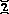)3 (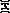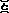)2 (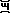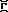)1 (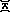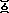)0 Go ahead and convert to Ethiopic numbers. 7) () + (3 * {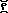}) () + (2 * {}) () + (1 * {}) () + (0 * {}) The subscripts now tell how many's we need. 8) () + (++) () + (+) () + () () + (0) 9) () + (+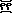) () + () () + () () + (0) Reduce as per=+10)Group... 11)Collect and we're done! Note! Except for when we use subscript ``0'' (the far right side) there is a rule that 1's in the 1's place are absorbed by an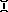,, oron the right. So if we changed the ``5'' in 2 to a ``1'' in the above; the reduction would go: as 3) 3 2 1 0 We'll add subscripts for book keeping. : : : : : : 9) () + (+) () + () () + () () + (0) Reduce as per=+10)Group...

The interesting consequence then is thatcan only appear in the one's place (the far right)!